Software Development

# Calculation of Mean in Individual Series | Formula of Mean

## What is Mean?

Mean is the sum of a set of numbers divided by the total number of values. It is also referred to as the average. For instance, if there are four items in a series, i.e. 2, 5, 8, 3, and 9. The simple arithmetic mean is (2 + 5 + 8 + 3 + 9) / 5 = 5.4.

### What is Individual Series?

The series in which the items are listed singly is known as Individual Series. In simple terms, a separate value of the measurement is given to each item. For example, if the height of 20 students in a class is given individually, then the resultant series will be an individual series.

### Mean in Individual Series:

The mean is calculated by adding up all the observations and dividing it by the total number of observations in the set. There are three ways to determine the arithmetic mean of an individual series:

1. Direct Method;
2. Short-Cut Method; and
3. Step Deviation Method

#### Example 1:

Find the average for the following data using Short-Cut Method: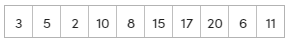#### Solution: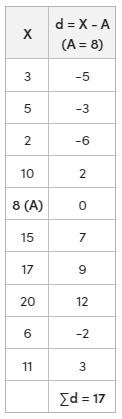Mean = 9.7

#### Example 2:

The Mean of a series with 5 items is 40, and the values of four items are 35, 10, 65, 50. Find out the missing 5th item.

#### Solution:In the given question, the following values are given:

X1 = 35, X2 = 10, X3 = 65, X4 = 50,= 40, and N = 5200 = 35 + 10 + 65 + 50 + X5

X5 = 200 – 160

Missing Value (X5 = 40)

#### Example 3:

The following table shows the marks obtained by 5 students out of 100 at a tuition center using direct method and step-deviation method.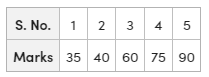#### Solution:

Direct Method: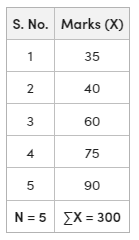Mean () = 60

Step-Deviation Method: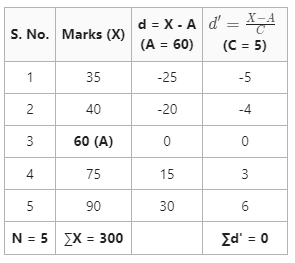Mean () = 60

Last Updated :
03 Aug, 2023

Like Article

Save Article